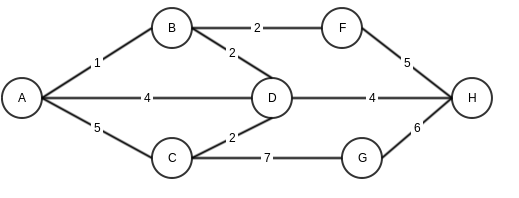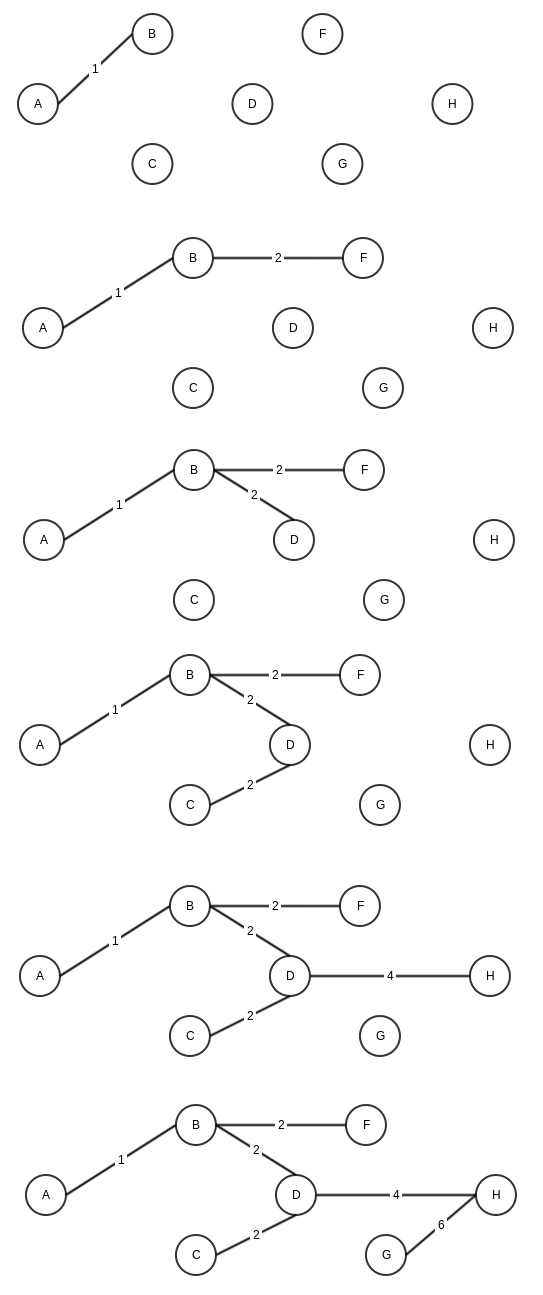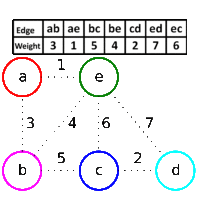# Kruskal’s Algorithm for Minimum spanning tree.

In last post I talked about Prim’s algorithm for minimum spanning tree.

Kruskal is another alorithm to solve same problem.

1. To start with, remove all edges from graph.
2. Add edges to a set S in sorted order with increasing order of weight.
3. Take an edge from set S (next minimum weight) and add to graph if (and only if) it is connecting non- cnnected nodes. Igore the edge if vertices are already connected.

So if I try to same problem for this graph.These will be the stepsAnother interesting depiction from wikipedia